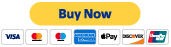### 1.      Information Theories

#### a)      This is one model for the measuring the number of facts of information surrounding a human over a given time, K(t).  It does this in a numerical fashion and considers neither the accuracy of the facts it counts, nor thoughts that are not facts.  For example, if a person made the statement, “I know bananas are blue”, it counts as something one knows whether it’s correct or not, but, “I wonder if I will ever visit outer space” does not count at all.  However, considering the examples, the model for this person would include and count the facts that they “don’t know that bananas are commonly labeled yellow”, and “I know I wonder if I will ever visit outer space”.  Hopefully this will be clearer after the next paragraph.

##### (1)         Typically, a human is not aware of the numerical value of the number facts it knows.  Similarly, it is not aware of the number of facts it does not know.  What then can be said about the relations between these values logically and mathematically?  It seems, that what we do or do not know, can in one model, be divided into 6 groups (A through F).  Each group breaks down further into the past, present, and future, when a sense of time is considered (number subscript).  Also, consider these other six groups at time t assigning them positive magnitudes: initial info (KIn), final info (KF), info gained or learned between the past and present (K1), info gained or learned between present and future (K2), info forgotten or lost between past and present (K3), and info to be forgotten or lost between the present and future (K4). Therefore, K1 + K2 - K3 - K4 = KNET, where KIn + KNet = KF.  This represents the net info between the past and future over the course of ones life.  Of course in many belief systems, at a life’s end K2 and K4 disappear, and the equation becomes K1 - K3 = KNet.  Finally, there is also KT, knowledge total, which is all there is to know, and KU, facts unknown, which is the difference between KT and KF (KT – KF = KU).  Using the present tense case to label each group heading is described following the next paragraph.

The sequence of these values in time must also be considered.  This is set such that time event 0 corresponds to KIn.  The letter name of a variable (A, B, C, etc) signifies its group.  The subscript after a variable distinguishes between members in a same group.

##### (3)   What you know (A)
###### (a)    What you knew (A1)
• A1(0) = 0

• A1(t) = A2(tn)  for any tn < t

• A1(t) = A3(tn)  for any tn < t

###### (b)   What you know (A2)
• A2(0) = KIn = KT(0) – KU(0) - KNet(0)

• A2(t) = KIn + K1(t) – K3(t)

• A2(t) + K1(tn) - K1(t) + K3(t) - K3(tn) = A2(tn)for tn > t

•

###### (c)    What you will know (A3)
• A3(0) = KIn + K2 (0) – K4 (0)

• A3(t) = A2(t) + K2 (t) – K4 (t) = KF(t)

•

##### (4)   What you know you know (B)
###### (e)    What you know you know (B5)
• B5(t) = A2(t)

##### (5)   What you don’t know (C)
###### (b)   What you don’t know (C2)
• C2(t) = KU(t)

##### (10)           Other comments at this time:  I can’t keep up with all the variables; comparing the mathematical equations to the verbal equivalents and then logically trying to verify validity.  If anyone skilled gets a hold of this, it would be great to see what a more complete model would look like.© 2017- 2020 by Thoughtfarm. Proudly created with Wix.com

This site was designed with the
.com
website builder. Create your website today.